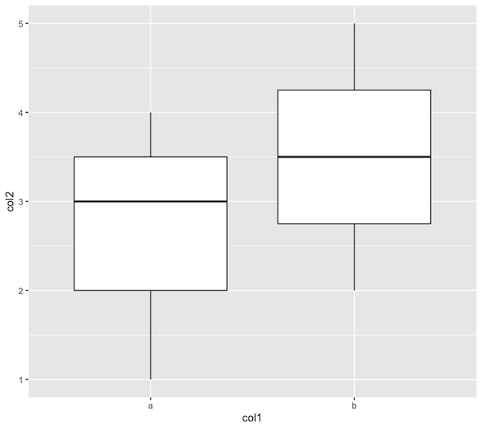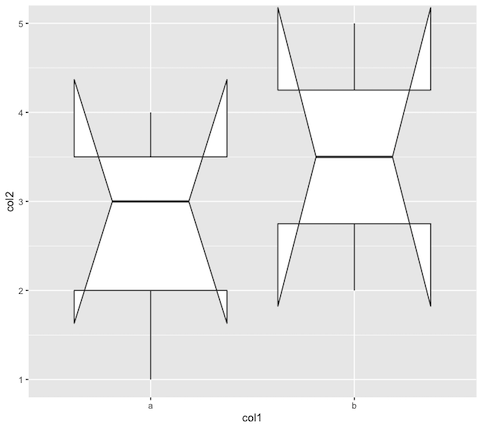GFG App
Open AppBrowser
Continue

# Adding Notches to Box Plots in R

In this article, we will study how to add a notch to box plot in R Programming Language.

The ggvis package in R is used to provide the data visualization. It is used to create visual interactive graphics tools for data plotting and representation. The package can be installed into the working space using the following command :

`install.packages("ggvis")`

The ggvis method in the ggvis package is used to start ggvis graphical window.

Syntax: ggvis( data , mp1, mp2.,)

Arguments :

• data – The dataset to plot
• mp1, mp2,.. – The map variables to plot

The ggplot() method is used to take as input an R object to plot the data points. It also takes the aesthetic mappings into consideration. The x and y coordinates can be plotted in the aesthetic mappings.

Syntax: ggplot (data-frame-obj , aes = )

Arguments :

• data-frame-obj – The data frame object
• aes – The aesthetic mappings to be specified

The geom_boxplot() method is used to construct a box plot in R. By default, the value of the notch parameter is “FALSE”.

## R

 `# installing the required libraries ` `library``(``"ggvis"``) ` ` `  `# creating a data frame ` `data_frame <- ``data.frame``(col1 = ``c``(``"a"``,``"b"``,``"a"``, ` `                                  ``"a"``,``"b"``), ` `                         ``col2 = ``c``(1:5)) ` `print``(``"Data Frame"``) ` `print``(data_frame) ` ` `  `# PLOTTING WITHOUT NOTCH  ` `ggplot``(data_frame, ``aes``(x = col1, y = col2)) + ` `  ``geom_boxplot``()`

Output

``` "Data Frame"
col1 col2
1    a    1
2    b    2
3    a    3
4    a    4
5    b    5```In order to add notch to the boxplot we can set the notch parameter to TRUE. It then adds notches to the boxplot.

## R

 `# installing the required libraries ` `library``(``"ggvis"``) ` ` `  `# creating a data frame ` `data_frame <- ``data.frame``(col1 = ``c``(``"a"``,``"b"``,``"a"``, ` `                                  ``"a"``,``"b"``), ` `                         ``col2 = ``c``(1:5)) ` `print``(``"Data Frame"``) ` `print``(data_frame) ` ` `  `# plotting with notch ` `ggplot``(data_frame, ``aes``(x = col1, ` `                       ``y = col2)) + ` `  ``geom_boxplot``(notch = ``TRUE``)`

Output

``` "Data Frame"
col1 col2
1    a    1
2    b    2
3    a    3
4    a    4
5    b    5```My Personal Notes arrow_drop_up